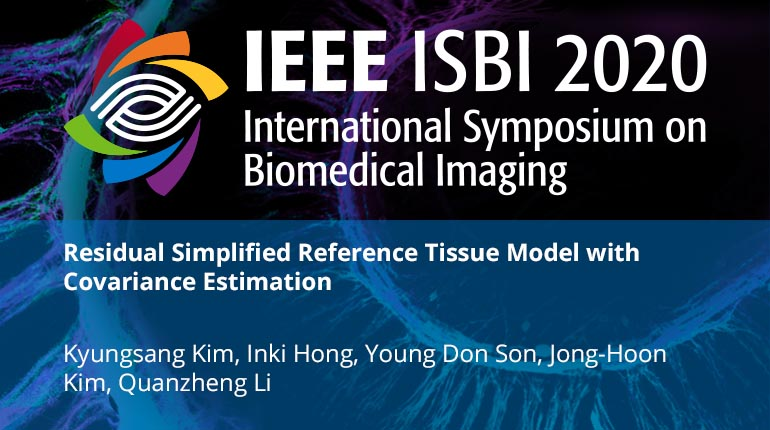This video program is a part of the Premium package:

### Residual Simplified Reference Tissue Model with Covariance Estimation

• IEEE MemberUS \$11.00
• Society MemberUS \$0.00
• IEEE Student MemberUS \$11.00
• Non-IEEE MemberUS \$15.00

# Residual Simplified Reference Tissue Model with Covariance Estimation

0 views
The simplified reference tissue model (SRTM) can robustly estimate binding potential (BP) without a measured arterial blood input function. Although a voxel-wise estimation of BP, so-called parametric image, is more useful than the region of interest (ROI) based estimation of BP, it is challenging to calculate the accurate parametric image due to lower signal-to-noise ratio (SNR) of dynamic PET images. To achieve reliable parametric imaging, temporal images are commonly smoothed prior to the kinetic parameter estimation, which degrades the resolution significantly. To address the problem, we propose a residual simplified reference tissue model (ResSRTM) using an approximate covariance matrix to robustly compute the parametric image with a high resolution. We define the residual dynamic data as full data except for each frame data, which has higher SNR and can achieve the accurate estimation of parametric image. Since dynamic images have correlations across temporal frames, we propose an approximate covariance matrix using neighbor voxels by assuming the noise statistics of neighbors are similar. In phantom simulation and real experiments, we demonstrate that the proposed method outperforms the conventional SRTM method.
The simplified reference tissue model (SRTM) can robustly estimate binding potential (BP) without a measured arterial blood input function. Although a voxel-wise estimation of BP, so-called parametric image, is more useful than the region of interest (ROI) based estimation of BP, it is challenging to calculate the accurate parametric image due to lower signal-to-noise ratio (SNR) of dynamic PET images. To achieve reliable parametric imaging, temporal images are commonly smoothed prior to the kinetic parameter estimation, which degrades the resolution significantly. To address the problem, we propose a residual simplified reference tissue model (ResSRTM) using an approximate covariance matrix to robustly compute the parametric image with a high resolution. We define the residual dynamic data as full data except for each frame data, which has higher SNR and can achieve the accurate estimation of parametric image. Since dynamic images have correlations across temporal frames, we propose an approximate covariance matrix using neighbor voxels by assuming the noise statistics of neighbors are similar. In phantom simulation and real experiments, we demonstrate that the proposed method outperforms the conventional SRTM method.